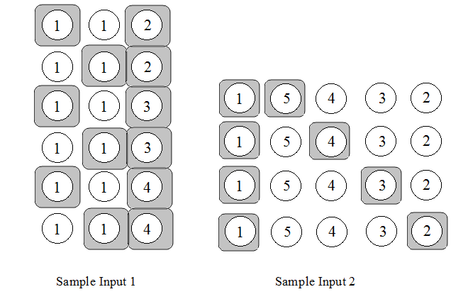# LIDSshovonshovoBACS Regional Programming...
Limits 1s, 512 MB

We all know about LIS (Longest Increasing Sub sequence). The task to find the length of the longest increasing sub sequence in a given array of integers is very easy. For example, the length of the LIS for $\{15, 27, 14, 38, 26, 55, 46, 65, 85\}$ is 6 and the longest increasing sub sequence is $\{15, 27, 38, 55, 65, 85\}$.

But, do we all know about LIDS? The task to find the length of the longest increasing digit sub sequence within an integer is known as LIDS. For example, length of LIDS for 1234 is 4, length of LIDS for 12234 is 4, length of LIDS for 456123 is 3. $\{7\}$, $\{1,4,9\}$, $\{5,9\}$ are some valid increasing digit sequence while $\{3,2\}$, $\{1,1\}$, $\{4, 9, 1\}$ are invalid.

You are given two integers x and y. You have to answer maximum length of LIDS between x and y inclusive and the number of different ways maximum LIDS can be formed. Two ways are considered different if the longest increasing digit sequence are not same or they are chosen from different position in an integer.

## Input

Input starts with an integer $T$ ($T ≤ 10000$), denoting the number of test cases. Each of the test cases consists of two space separated integers $x$ and $y$ denoting the range.

Easy subtask: $1 ≤x ≤y <1000$

Medium subtask: $1≤x≤y≤1000,000,000$ and $(y-x) ≤1000$

Hard subtask: $1≤x≤y≤1000,000,000$

Digits are: 0, 1, 2, 3, 4, 5, 6, 7, 8 and 9.

## Output

For each of the test cases, you need to print one line of output. The output for each test case starts with the test case number, followed by Maximum Length of LIDS and the number of ways LIDS can be formed. You must output as it is given in the sample output section.

## Sample

InputOutput
2
111 114
15432  15432

Case 1: 2 6
Case 2: 2 4


For First Sample, Length LIDS of 111 is 1 and LDIS is $\{1\}$. Length LIDS of 112 is 2 and LIDS is $\{1, 2\}$. Length LIDS of 113 is 2 and LIDS is $\{1, 3\}$. Length LIDS of 114 is 2 and LIDS is $\{1,4\}$. So, Maximum Length of LIDS is 2. And It can be formed in 6 ways.

For Second Sample, there is exactly one number. Length LIDS of 15432 is 2. We can see, there are four different possible solutions. They are: $\{1, 5\}$, $\{1, 4\}$, $\{1, 3\}$ and $\{1, 2\}$.uDebug

### Submit